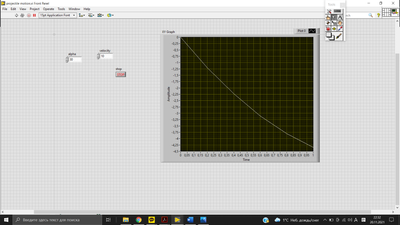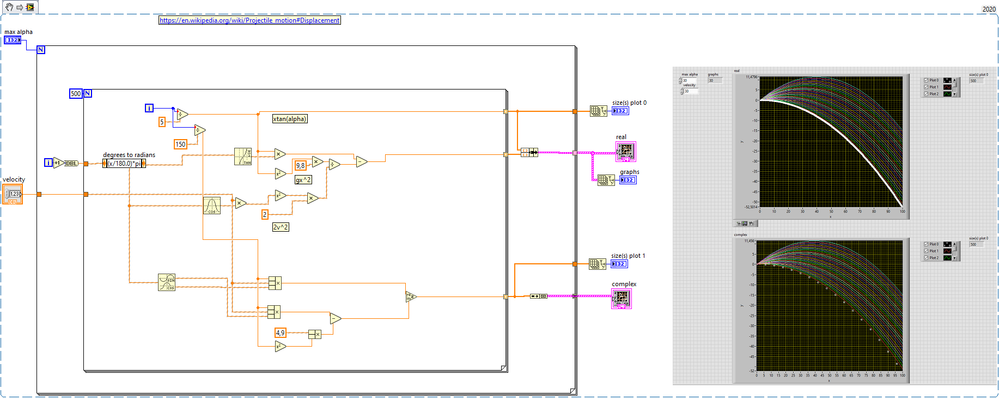# LabVIEW

cancel
Showing results for
Did you mean:

## Projectile motion

I am trying to create the simulation of a projectile motion in Labview. Here is vi I am using.  The formula for y I am using is this : y = (tan θ0)x – gx2/2(v0cosθ0)2

I am using case structure to allow negative values of angles to exist too. I read here in community that for negative angles the formula needs to have + instead of - .  Parabola comes out okay for almost every angle, however for 30 degree it comes out like this:I suspect it is because x*tan(alpha) is smaller than the second part of the formula, therefore negative values come out. 60 degrees and 45 are okay. How can I get a simulation for 30 degrees?

Message 1 of 9
(720 Views)

## Re: Projectile motion

First of all, note that the trigonometric functions want radians (full circle is 2pi, not 360), so a value of "30" corresponds to quite a few turns. Make sure to convert it first!

It would be so much simpler to calculate the evolution of  x and y independently (each initialized according to angle and magnitude). Now the speed in x is constant and the speed in y is a simple acceleration. (you can even add a air friction term later if needed). No tan needed.

Even easier, do things using complex numbers!LabVIEW Champion.Message 2 of 9
(717 Views)

## Re: Projectile motion

It seems ironic to me that "simpler" is "complex".

Bill(Mid-Level minion.)
My support system ensures that I don't look totally incompetent.
Proud to say that I've progressed beyond knowing just enough to be dangerous. I now know enough to know that I have no clue about anything at all.
Humble author of the CLAD Nugget.
Message 3 of 9
(692 Views)

## Re: Projectile motion

Bill,

"Complex" just isn't "Real", it is also "Imaginary" (at the same time ...).  Still, it does make for a "simpler" (in some mathematical sense) mapping of a 2-Dimensional space than do "real" numbers (which take Two to Tango ...).

Bob Schor

Message 4 of 9
(688 Views)

## Re: Projectile motion

I just read my previous response to my wife, and told her it had 4 Kudos.  She said I should say "If you like this response, don't thank me, thank i ".  But that would just be silly ...

Bob Schor

Message 5 of 9
(559 Views)

## Re: Projectile motion

4 kudos is just under-rated, these puns are worth being made historic so that someone in the future can quote it as "by Bob Schor"

-Santhosh
Semiconductor Validation & Production Test
Soliton Technologies
NI CLD, CTD
LabVIEW + TestStand + TestStand Semiconductor Module (2013 - 2020)
NI STS for Mixed signal and RF
Message 6 of 9
(553 Views)

## Re: Projectile motion

that was funMessage 7 of 9
(503 Views)

## Re: Projectile motion

@Bob_Schor wrote:

Bill,

"Complex" just isn't "Real", it is also "Imaginary" (at the same time ...).  Still, it does make for a "simpler" (in some mathematical sense) mapping of a 2-Dimensional space than do "real" numbers (which take Two to Tango ...).

Bob Schor

There are many cases where using complex numbers simplifies the math.

In circuit analysis, Ohm's law doesn't work for AC, if you use real numbers.  But if you use complex voltage, complex current, and complex impedance, then Ohm's law does work and you can do circuit analysis without trig.

"If you weren't supposed to push it, it wouldn't be a button."
Message 8 of 9
(484 Views)

## Re: Projectile motion

@paul_cardinale wrote:
There are many cases where using complex numbers simplifies the math.

Here's my quick attempt using complex only (no explicit trigonometry!). I am sure it can be polished up a bit. 😄LabVIEW Champion.Message 9 of 9
(442 Views)